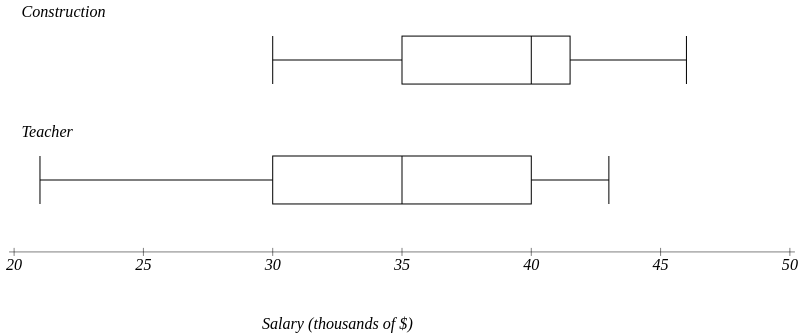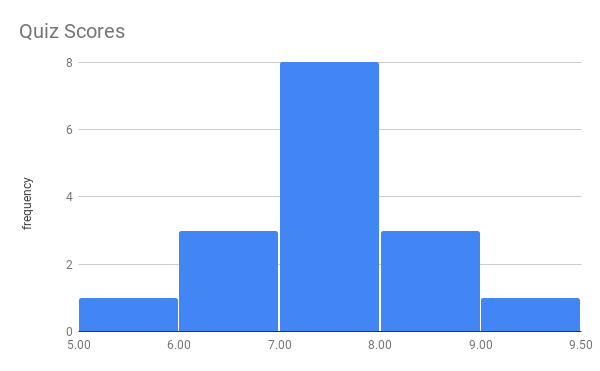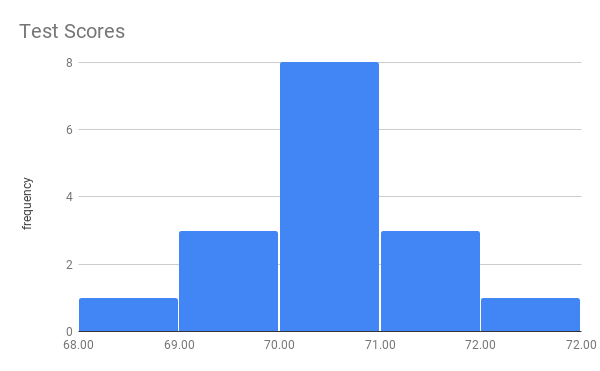Statistics Notes on Describing Data
Spring 2019

## Chapter 2 Descriptive Statistics

### Part 1: Organizing Data

#### § 2.1 Stem-and-Leaf Graphs (Stemplots), Line Graphs, and Bar Graphs

• Stem and Leaf Graph (aka stem plot)
Consider the final significant digit
Example: Age at Inauguration of 20th Century US Presidents
42, 51, 56, 55, 51, 54, 51, 60, 62, 43, 55, 56, 61, 52, 69, 64, 46, 54, 47, 70
Order the values:
42, 43, 46, 47, 51, 51, 51, 52, 54, 54, 55, 55, 56, 56, 60, 61, 62, 64, 69, 70
Age at Inauguration of 20th Century US Presidents
Stem | Leaves
4  | 2, 3, 6, 7
5  | 1, 1, 1, 2, 4, 4, 5, 5, 6, 6
6  | 0, 1, 2, 4, 9
7  | 0

• Line Graph
Could be an alternative to a bar/column graph, but is better for data over time.
• Bar Graph
vertical or horizontal bars representing some quantity.
aka column chart
Comparative Bar graphs?
show examples with too high a growth factor.

#### § 2.3 Measures of the Location of the Data § 2.4 Box Plots

• Percentiles with Quantitative Data
• Percentile
• Percentile location, aka data index/position
• Percentile value
• Examples
• P10 is the 10th percentile, that is, the position in the data set were 10% of the data is at or below that point.
• Suppose we have a sample of size n=20. Then the position of P10 is 0.10*(20+1)=2.1, or the number in the 2nd position when ranked.
• Consider the Presidents ages:
Example: Age at Inauguration of 20th Century US Presidents
42, 43, 46, 47, 51, 51, 51, 52, 54, 54, 55, 55, 56, 56, 60, 61, 62, 64, 69, 70
So, P10=43
According to MS Excel or Google Sheets, P10 = PERCENTILE.INC(data,0.1) = 45.7
Close, but different from my method. There are other ways to identify percentile values that will be close to but different from each of these.
Oh, well.
• Quartile
• percentiles
• locators
• 5 different quartiles
• Quartile vs Quarter
• Interpretaion
Statistic Construction Teacher
min 30 21
Q1 35 30
m 40 35
Q3 42 40
Max 46 43• 5 number summary and the Box Plot
• Extended InterQuartile Range
aka Outlier Fences, lower and upper
Lower Fence = Q_1-1.5*IQR
Upper Fence = Q_3+1.5*IQR
These values fence in "usual" data, hence give us a measure for outliers, aka, "unusual" data.

#### § 2.5 Measures of Center

• bar(x) = Mean = Arithmetic Mean = Average = Sum / Count
spreadsheet function =AVERAGE(data)
• m = Median = middle when ranked
locator = (n+1)/2
spreadsheet function =MEDIAN(data)
• Mode = most frequently occurring = good for qualitative data, among others
• The Law of Large Numbers and the Mean
Larger samples and/or repeated sampling will get barx closer to mu.
• Sampling Distributions and Statistic of a Sampling Distribution
A sampling distribution is a distribution of samples.
• Calculating the Mean of Grouped Frequency Tables
Example 1
Example 2
Scores Frequencies
68 1
69 3
70 8
71 3
72 1
• Interpreting the mean
• Context
• The average human body temperature is 97.6.
OK.
• The average U.S. household has 2.53 people. (ref)
Wait. How can you have half of a person?
Right. OK.
The average U.S. household has between 2 and 3 people.
Thanks

#### § 2.6 Measures of Skewness/Center

The skew is to the tail.

• Symmetrical
mean ~~ median• Skewed left
mean < median• Skewed right
mean > median#### § 2.7 Measures of Spread

• s = Standard Deviation of a Sample = sqrt((sum (x-barx)^2)/(n-1))
spreadsheet function =STDEV(data)
• sigma = Standard Deviation of a Population
• Range = max - min
• IQR = InterQuartile Range = Q_3-Q_1
• Examples: Mortality Rate by Country, in Sheets, Docs, StatKey, other
• Counting StDs and z-scores
• Coefficient of Variation = CV = s/(barx)*100%
This is a measure of the standard deviation as a percentage of the mean.
What exactly does it mean to have a "large" standard deviation or a "small" standard deviation?
A StDev of 1 is large for a mean of 3, but small for a mean of 80. The CV can help to illustrate this.
Additionally, you can't compare the StDev of two samples directly unless the samples have the same mean.
So, the CV allows us to compare the StDevs of two samples with different means.
vsbarx=7, s~~1, and CV~~1/7~~14.3%barx=70, s~~1, and CV~~1/70~~1.43%
Two Sample Example
• Can standard deviation help us find outliers? Unusual values?
We consider the extreme 5% of the data, this is often outside 2 standard deviations from the mean.